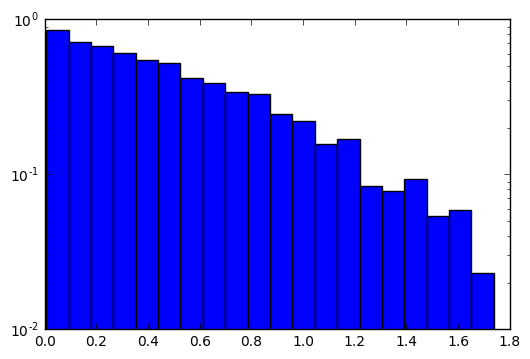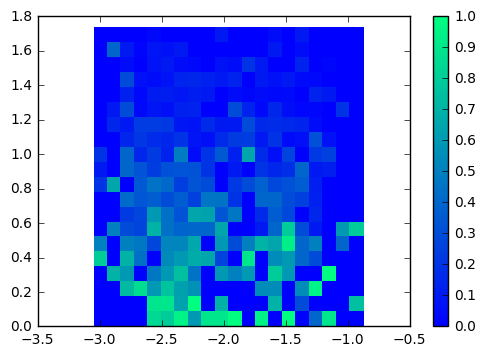# Committor Simulation and Analysis for Alanine Dipeptide

This sequence of notebooks provides an example of how to calculate the committor for a real biomolecular system (alanine dipeptide).

The first notebook deals with selecting the snapshots to use as starting points for the committor. In this particular case, we didn’t care about the statistics of those points, so we select them directly from storage. This is because these points were being harvested as part of an approach to estimate the committor at any given point. If you need the statistics of those points within the TPS ensemble, e.g., for the transition state ensemble, then you should select your points differently (by looping over storage.steps and taking the active sample set).

The second notebook runs the committor simulation. The third notebook prepares the analysis data in a way that was needed for this particular project, where each “shot” of the committor was treated as a separate “experiment.” While this approach is not normally needed, if you already have data that can easily be put into this format, the fourth notebook shows how it can be used to construct a ShootingPointAnalysis object. That notebook also proceeds to demonstrate the kinds of analysis that object can perform.

1_select_snapshots

# Select Snapshots¶

This notebook will take an old (and large) TPS simulation file, select some snapshots to use as input data.

Note: this first version is quick and dirty. There might be some points to consider to select better snapshots. But this is just intended to get initial data to our colleagues.

In :
import openpathsampling as paths
import random

In :
%%time
storage = paths.Storage("alanine_dipeptide_tps.nc", "r")

CPU times: user 20.7 s, sys: 6.48 s, total: 27.2 s
Wall time: 2min 10s

In :
print storage.file_size_str

17.95GB

In :
n_snapshots = len(storage.snapshots)
print n_snapshots

946650

In :
stateA = storage.volumes['C_7eq']
stateB = storage.volumes['alpha_R']


Now we do the main calculation: every snapshot must not be in a state, and we never re-use a snapshot. (In other words, randomly chosen without replacement.)

In addition, OPS snapshots are always listed in pairs, with velocities reversed. (The data is only stored once, but both can be accessed directly from the snapshot storage.) Because of this, we'll make sure we only take the even-numbered snapshots.

In :
%%time
snapshots = []
while len(snapshots) < 1000:
random_choice = random.randint(0, (n_snapshots/2)-1)
snap = storage.snapshots[random_choice*2]
if not stateA(snap) and not stateB(snap) and snap not in snapshots:
snapshots.append(snap)

CPU times: user 16.4 s, sys: 452 ms, total: 16.8 s
Wall time: 1min 1s

In :
new_store = paths.Storage("snapshots.nc", "w")

In :
new_store.save(snapshots);

In :
# save the old engine because we'll re-use its topology later
new_store.save(storage.engines);

In :
new_store.sync()
new_store.close()


2_committor_simulation

# Committor Simulation¶

In :
import openpathsampling as paths
import mdtraj as md
import numpy as np
from simtk import unit

In :
input_storage = paths.Storage("snapshots.nc", "r")

In :
print input_storage.file_size_str
print len(input_storage.snapshots)

42.89MB
2000

In :
# 2000 instead of 1000 because reversed snapshots are automatically counted
snapshots_to_run = input_storage.snapshots[::2]

In :
# conveniently, we saved the engine; have to re-do everything else
engine = input_storage.engines

# set up the collective variables for our states
phi = paths.MDTrajFunctionCV(name="phi",
f=md.compute_dihedrals,
topology=engine.topology,
indices=[[4, 6, 8, 14]])
psi = paths.MDTrajFunctionCV(name="psi",
f=md.compute_dihedrals,
topology=engine.topology,
indices=[[6, 8, 14, 16]])

In :
# define our states
deg = 180.0/np.pi
C_7eq = (paths.PeriodicCVDefinedVolume(phi, lambda_min=-180/deg, lambda_max=0/deg,
period_min=-np.pi, period_max=np.pi) &
paths.PeriodicCVDefinedVolume(psi, lambda_min=100/deg, lambda_max=200/deg,
period_min=-np.pi, period_max=np.pi)
).named("C_7eq")
# similarly, without bothering with the labels:
alpha_R = (paths.PeriodicCVDefinedVolume(phi, -180/deg, 0/deg, -np.pi, np.pi) &
paths.PeriodicCVDefinedVolume(psi, -100/deg, 0/deg, -np.pi, np.pi)).named("alpha_R")

In :
# OpenMM requires everything to have units
# beta = 1.0 / (k_B T)
temperature = 300.0 * unit.kelvin
beta = 1.0 / (temperature * unit.BOLTZMANN_CONSTANT_kB)

In :
randomizer = paths.RandomVelocities(beta=beta, engine=engine)

In :
output_storage = paths.Storage("committor_simulation.nc", "w")

In :
simulation = paths.CommittorSimulation(storage=output_storage,
engine=engine,
states=[C_7eq, alpha_R],
randomizer=randomizer,
initial_snapshots=snapshots_to_run)

In :
simulation.run(n_per_snapshot=10)

Working on snapshot 1000 / 1000; shot 10 / 10
In [ ]:



3_committor_analysis

# Committor Analysis¶

(Note: some of this requires OpenPathSampling 0.9.1 or later)

The particular approach used in this notebook is to save each shot as a separate "experiment," where each of the experiements is a tuple matching initial snapshot to final state. That was a peculiarity of the project from which this example originated. Typically, you could just go directly to the analysis of the shooting points. However, if you already have data from elsewhere that can be put into this format, the next notebook shows you how you can build a committor analysis from such a list.

In :
import openpathsampling as paths

In :
%%time
simulation_storage = paths.AnalysisStorage("committor_simulation.nc")
C_7eq = simulation_storage.volumes['C_7eq']
alpha_R = simulation_storage.volumes['alpha_R']

CPU times: user 1min 44s, sys: 29.6 s, total: 2min 14s
Wall time: 48min 2s

In :
analyzer = paths.ShootingPointAnalysis(steps=None, states=[C_7eq, alpha_R])

In :
%%time
# the shooting point snapshot for each
shooting_pts = [analyzer.step_key(step) for step in simulation_storage.steps]

CPU times: user 57.6 s, sys: 1.45 s, total: 59.1 s
Wall time: 2min 29s

In :
%%time
# get the final states from each partial trajectory
final_states_list = [analyzer.analyze_single_step(step) for step in simulation_storage.steps]

CPU times: user 4min 54s, sys: 4.4 s, total: 4min 59s
Wall time: 11min 6s

In :
# check that there's only one state per item in that list
for f in final_states_list:
assert len(f) == 1
# flatten the list
final_states = [f for f in final_states_list]

In :
experiments = zip(shooting_pts, final_states)

In :
output_storage = paths.Storage("committor_results.nc", "w")

In :
output_storage.save(C_7eq)
output_storage.save(alpha_R)
output_storage.tag['experiments'] = experiments

In :
output_storage.sync()
output_storage.close()


4_analysis_help

# Analysis help¶

This covers stuff that you will need to know in order to use the committor_results.nc file.

In :
%matplotlib inline
import matplotlib.pyplot as plt
import openpathsampling as paths
import numpy as np
import pandas as pd
pd.options.display.max_rows = 10

In :
storage = paths.Storage("committor_results.nc", "r")

In :
phi = storage.cvs['phi']
psi = storage.cvs['psi']

In :
%%time
C_7eq = storage.volumes['C_7eq']
alpha_R = storage.volumes['alpha_R']
experiments = storage.tag['experiments']

CPU times: user 49.6 s, sys: 239 ms, total: 49.8 s
Wall time: 51.4 s


The experiments object is a list of tuples (snapshot, final_state). Each snapshot is an OPS snapshot object (a point in phase space), and the final_state is either the C_7eq object or the alpha_R object.

## Directly obtaining a committor analysis¶

As it happens, experiments is in precisely the correct format to be used in one of the approaches to constructing a committor analysis.

This section requires OpenPathSampling 0.9.1 or later.

In :
%%time
committor_analyzer = paths.ShootingPointAnalysis.from_individual_runs(experiments)

CPU times: user 44 s, sys: 143 ms, total: 44.2 s
Wall time: 49.1 s


Before going further, let's talk a little bit about the implementation of the ShootingPointAnalysis object. The main thing to understand is that the purpose of that object is to histogram according to configuration. The first snapshot encountered is kept as a representative of that configuration.

So whereas there are 10000 snapshots in experiments (containing the full data, including velocities), there are only 1000 entries in the committor_analyzer (because, in this data set, I ran 1000 snapshots with 10 shots each.)

### Per-configuration results¶

The .to_pandas() function creates a pandas table with configurations as the index, the final states as columns, and the number of times that configuration led to that final state as entries. With no argument, to_pandas() using the an integer for each configuration.

In :
committor_analyzer.to_pandas()

Out:
C_7eq alpha_R
0 8.0 2.0
1 5.0 5.0
2 9.0 1.0
3 9.0 1.0
4 10.0 NaN
... ... ...
995 3.0 7.0
996 4.0 6.0
997 9.0 1.0
998 8.0 2.0
999 8.0 2.0

1000 rows × 2 columns

You can also pass it a function that takes a snapshot and returns a (hashable) value. That value will be used for the index. These collective variables return numpy arrays, so we need to cast the 1D array to a float.

In :
psi_hash = lambda x : float(psi(x))
committor_analyzer.to_pandas(label_function=psi_hash)

Out:
C_7eq alpha_R
0.824873 8.0 2.0
0.576733 5.0 5.0
0.521896 9.0 1.0
0.444523 9.0 1.0
0.977096 10.0 NaN
... ... ...
0.419521 3.0 7.0
0.353020 4.0 6.0
1.492791 9.0 1.0
1.054834 8.0 2.0
1.254955 8.0 2.0

1000 rows × 2 columns

You can also directly obtain the committor as a dictionary of (representative) snapshot to committor value. The committor here is defines as the probability of ending in a given state, so you must give the state.

In :
committor = committor_analyzer.committor(alpha_R)

In :
# show the first 10 values
{k: committor[k] for k in committor.keys()[:10]}

Out:
{<openpathsampling.engines.openmm.snapshot.Snapshot at 0x10f116b90>: 0.0,
<openpathsampling.engines.openmm.snapshot.Snapshot at 0x10f192f50>: 0.4,
<openpathsampling.engines.openmm.snapshot.Snapshot at 0x10f31a990>: 0.0,
<openpathsampling.engines.openmm.snapshot.Snapshot at 0x10f34ef10>: 0.3,
<openpathsampling.engines.openmm.snapshot.Snapshot at 0x10f5c13d0>: 0.3,
<openpathsampling.engines.openmm.snapshot.Snapshot at 0x10f9e1950>: 0.0,
<openpathsampling.engines.openmm.snapshot.Snapshot at 0x10fd170d0>: 0.3,
<openpathsampling.engines.openmm.snapshot.Snapshot at 0x1100f4c50>: 0.1,
<openpathsampling.engines.openmm.snapshot.Snapshot at 0x1101f7410>: 0.2,
<openpathsampling.engines.openmm.snapshot.Snapshot at 0x1103bd390>: 0.0}

### Committor histogram in 1D¶

In :
hist1D, bins = committor_analyzer.committor_histogram(psi_hash, alpha_R, bins=20)

In :
bin_widths = [bins[i+1]-bins[i] for i in range(len(bins)-1)]
plt.bar(left=bins[:-1], height=hist1D, width=bin_widths, log=True);### Committor histogram in 2D¶

In :
ramachandran_hash = lambda x : (float(phi(x)), float(psi(x)))
hist2D, bins_phi, bins_psi = committor_analyzer.committor_histogram(ramachandran_hash, alpha_R, bins=20)

In :
# not the best, since it doesn't distinguish NaNs, but that's just a matter of plotting
plt.pcolor(bins_phi, bins_psi, hist2D.T, cmap="winter")
plt.clim(0.0, 1.0)
plt.colorbar();## Obtaining information from the snapshots¶

The information committor_results.nc should be everything you could want, including initial velocities for every system. In principle, you'll mainly access that information using collective variables (see documentation on using MDTraj to create OPS collective variables). However, you may decide to access that information directly, so here's how you do that.

In :
# let's take the first shooting point snapshot
# experiments[N] gives shooting snapshot for experiment N
snapshot = experiments


OpenMM-based objects come with units. So snapshot.coordinates is a unitted value. This can be annoying in analysis, so we have a convenience snapshot.xyz to get the version without units.

In :
snapshot.coordinates

Out:
Quantity(value=array([[ 0.2793301 , -0.12214785, -0.202672  ],
[ 0.2449614 , -0.05111128, -0.1274817 ],
[ 0.33534443, -0.01180077, -0.08093405],
...,
[-1.46748817, -0.68787634,  0.40931037],
[-1.55375791, -0.72757316,  0.42131069],
[-1.45325804, -0.68954408,  0.31466872]], dtype=float32), unit=nanometer)
In :
snapshot.xyz

Out:
array([[ 0.2793301 , -0.12214785, -0.202672  ],
[ 0.2449614 , -0.05111128, -0.1274817 ],
[ 0.33534443, -0.01180077, -0.08093405],
...,
[-1.46748817, -0.68787634,  0.40931037],
[-1.55375791, -0.72757316,  0.42131069],
[-1.45325804, -0.68954408,  0.31466872]], dtype=float32)

For velocities, we don't have the convenience function, but if you want to remove units from velocities you can do so with velocity / velocity.unit.

In :
snapshot.velocities

Out:
Quantity(value=array([[ -3.21658134e-01,  -1.10456896e+00,   7.57985592e-01],
[ -2.63174623e-01,   7.86199495e-02,  -5.87066524e-02],
[ -4.26795304e-01,   3.19573097e-03,  -2.02318802e-01],
...,
[  2.87866861e-01,   5.24361193e-01,   2.04794154e-01],
[ -3.03164870e-01,   3.03924894e+00,  -1.35492578e-01],
[ -1.56592965e+00,  -4.29962158e+00,  -5.62753141e-01]], dtype=float32), unit=nanometer/picosecond)
In :
snapshot.velocities / snapshot.velocities.unit

Out:
array([[ -3.21658134e-01,  -1.10456896e+00,   7.57985592e-01],
[ -2.63174623e-01,   7.86199495e-02,  -5.87066524e-02],
[ -4.26795304e-01,   3.19573097e-03,  -2.02318802e-01],
...,
[  2.87866861e-01,   5.24361193e-01,   2.04794154e-01],
[ -3.03164870e-01,   3.03924894e+00,  -1.35492578e-01],
[ -1.56592965e+00,  -4.29962158e+00,  -5.62753141e-01]], dtype=float32)

Note that snapshots include coordinates and velocities. We have several sets of initial velocities for each initial snapshot. Taking the second shooting snapshot and comparing coordinates and velocities:

In :
snapshot2 = experiments

In :
np.all(snapshot.coordinates == snapshot2.coordinates)

Out:
True
In :
np.any(snapshot.velocities == snapshot2.velocities)

Out:
False